# In and out

The empty tank is filled in 12 minutes and empty in 16 minutes. How long does it take to fill if we forgot to close the drain?
If the tank has 1000 l.

Result

t =  48 min

#### Solution:

t*(1/12 - 1/16)=1

t = 48

t = 48

Calculated by our simple equation calculator.

Leave us a comment of example and its solution (i.e. if it is still somewhat unclear...):

Showing 0 comments:Be the first to comment!#### To solve this example are needed these knowledge from mathematics:

Looking for help with calculating harmonic mean? Looking for a statistical calculator? Do you have a linear equation or system of equations and looking for its solution? Or do you have quadratic equation?

## Next similar examples:

1. Two pumps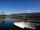The first pump fills the tank in 24 hours second pump in another 40 hours. For how long will take fill the tank if operate both pumps at the same time?
2. Two tributaries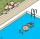Two tributaries of the pool fill it in 10 hours. One of the tributaries would fill 15 hours. How long would fill the first tributary?
3. Together 3If Sally can paint a house in 4 hours, and John can paint the same house in 6 hours, how long will it take for both of them to paint the house together?
4. Two looms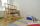On two different looms with different performance can be make required quantity of textile in 6 hours when both work together. On the first machine this quantity of the textile can make in 10 hours. How long should take make same quantity of textile on a s
5. Painters 4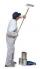One painter will do the job for 12 days. After a 4 1/3 day he comes second to help him and complete work together for 8 days. How long would work the second painter himself?
6. NovakNovak needed to dig up three of the same pit in the garden. The first pit dug father alone for 15 hours. His second dig son helped him and it did that in six hours. The third pit dug son himself. How long it took him?
7. Apprentice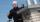Worker and apprentice execute the work for 6 hours. Worker performs himself in 10 hours. How long do the work of an apprentice?
8. Two diggers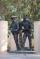There are two diggers. One digger digs a pit 46 hours second gidding 2 times faster. a) how long took to dig a pit second digger b) how long took dig together
9. The factoryThe factory z can complete an order in 12 days, factory p in 18 days. For how many days does an order completed when the first two days work only the factory z and then both factories?
10. GivenGiven 2x =0.125 find the value of x
11. Simplify 2Simplify expression: 5ab-7+3ba-9
12. Simple equationSolve for x: 3(x + 2) = x - 18
13. Workers 9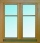If two workers fix two windows in an hour how many windows can 18 workers fix in the same two windows?
14. SoldierAn experienced soldier digs a good trench in 30 hours. Two soldiers dug the same trench each 40 hours. How many hours will the ditch dig all three together?
15. Holidays - on poolChildren's tickets to the swimming pool stands x € for an adult is € 2 more expensive. There was m children in the swimming pool and adults three times less. How many euros make treasurer for pool entry?
16. Fifth of the numberThe fifth of the number is by 24 less than that number. What is the number?
17. Working togetherOne painter paint the nursery in 10 days, the second in 8 days. How many days will the nursery be painted if they work together?